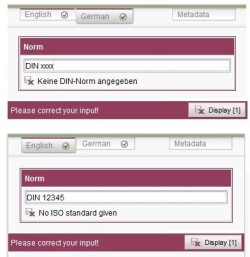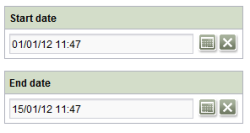## <IF/> Designing preconditions for rule execution

A validation by default (see page Time of execution) takes place directly during the entry of a value into an input component. This means that the rule is performed every time the editor presses a key.

This behavior is not desired in all cases. In case of more complex requests or a very extensive rule definition, it might make sense to define a precondition for the execution of the rule. (As an alternative, a special time of execution can be defined, see page Time of execution)

Preconditions are defined within an <IF/> section. This section must be defined before the tags for a value determination.

In the process, the following applies for the definition of a precondition:

• A precondition can be optionally defined in a rule. (If no precondition is defined, the rule is always performed.)
• A Boolean value is determined through a precondition. If the precondition is fulfilled (TRUE), the subsequent rule sections are performed; if the precondition is not fulfilled (FALSE), they are not performed.
• The precondition always refers to a certain property of the form element or of the form (“was the form opened in the Content Store?”).
For this purpose, the specification of a <PROPERTY/> tag within the <IF/> section is necessary. Through additional values can be derived. The value of the input component, for example, can be checked against certain regular expressions or against a comparison value.

## Examples

### Example 1) Language-dependent validation using a preconditionThe definition of a precondition is ideally suited for a language-dependent validation. In the following example, only a certain branch of the rule is performed depending on the currently processed editing language. The input component “st_standard”, for which the rule is defined, permits the language-dependent specification of a DIN standard (for German (“DE”)) or an ISO standard (for English (“EN”)). Using the definition of a precondition, the rule checks in which language the form is being processed by the editor (language-dependent validation).

During the processing of the editorial language “DE”, the following precondition is considered to be fulfilled:

` <IF> <EQUAL> <PROPERTY source="#global" name="LANG"/> <TEXT>DE</TEXT> </EQUAL> </IF>`

In this way, the subsequent branches of the rule that check whether the entry of the editor corresponds with a correct DIN standard format using a regular expression are performed. As long as the condition is not fulfilled (FALSE), the instructions are executed within the <VALIDATION/> section:

`...<WITH>    <MATCHES regex="^DIN\ \d\d*\$">        <PROPERTY source="st_standard" name="VALUE"/>    </MATCHES></WITH><DO>    <VALIDATION scope="SAVE">        <PROPERTY source="st_standard" name="VALID"/>        <MESSAGE lang="*" text="No DIN standard given"/>        <MESSAGE lang="DE" text="Keine DIN-Norm angegeben"/>    </VALIDATION></DO>...`

For additional editorial languages, the rule definition is expanded by a rule that checks whether the entry of the editor follows a correct ISO standard format using a regular expression according to a fulfilled precondition:

`...<RULE>    <IF>        <NOT>            <EQUAL>                <PROPERTY name="LANG" source="#global"/>                <TEXT>DE</TEXT>            </EQUAL>        </NOT>    </IF>    <WITH>        <MATCHES regex="^ISO\ \d\d*\$">            <PROPERTY name="VALUE" source="st_standard"/>        </MATCHES>    </WITH>    <DO>        <VALIDATION scope="SAVE">                <PROPERTY name="VALID" source="st_standard"/>                <MESSAGE lang="*" text="No ISO standard given"/>                <MESSAGE lang="DE" text="Keine ISO-Norm angegeben"/>            </VALIDATION>        </DO></RULE>...`

### Example 2) Preventing the exceeding of values using a preconditionIn an additional form, there is one entry field each for a start date (“st_startDate”) and an end data (“st_endDate”). Using a dynamic form, an end data is automatically calculated and assumed in the input component “st_endDate” when the editor enters a start date (see the Calculate Date example).

In the process, an end date already entered by the editor should not be overwritten by the rule. To guarantee that the rule is performed only if the “st_endDate” input component is not already fulfilled, a precondition is defined:

`<IF>    <PROPERTY name="EMPTY" source="st_endDate"/></IF>`

The following sections of the rule—that is, the calculation of a new date and the assumption of the new value in the input component “st_endDate”—are not fulfilled until this precondition is fulfilled.

`<RULE>    <IF>        <PROPERTY name="EMPTY" source="st_endDate"/>    </IF>    <WITH>        <ADD value="2 weeks">            <PROPERTY name="VALUE" source="st_startDate"/>        </ADD>    </WITH>    <DO>        <PROPERTY name="VALUE" source="st_endDate"/>    </DO></RULE>`